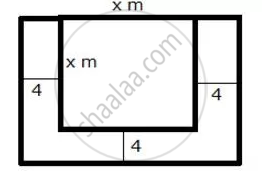Share

# A Square Lawn is Bounded on Three Sides by a Path 4 M Wide. If the Area of the Path is 7/8 that of the Lawn, Find the Dimensions of the Lawn. - Mathematics

Course

#### Question

A square lawn is bounded on three sides by a path 4 m wide. If the area of the path is 7/8 that of the lawn, find the dimensions of the lawn.

#### Solution

Let the side of the square lawn be x m.
Area of the square lawn = x2 m2
The square lawn is bounded on three sides by a path which is 4 m wide.Area of outer rectangle = (x + 4) (x + 8) = x2 + 12x + 32
Area of path = x2 + 12x + 32 – x2 = 12x + 32
From the given information, we have:

12x + 32 = 7/8 x^2

96x + 256 = 7x^2

7x^2 - 96x - 256 = 0

7x^2 - 112x + 16x - 256 = 0

7x(x - 16) + 16(x - 16) = 0

(x - 16)(7x + 16) = 0

x = 16, (-16)/7

Since, x cannot be negative. So, x = 16 m.
Thus, each side of the square lawn is 16 m.

Is there an error in this question or solution?